# Decorating graphics with Matplotlib

Standard

In the last entry, we saw a brief introduction to Matplotlib by drawing three basic graphic types (a line plot, a bar chart and pie chart). But the resulting graphics were arguably unattractive.

In the current scenario where Big Data is growing in popularity outside of the Data Science community, presentation often plays a key role in a successful results dissemination. Therefore, decoration of graphics should not be treated as a minor aspect.
In this blog entry we will draw the graphics of the last entry and decorate them.

We start with the line plot. We won’t review the whole code, just the new stuff. Due to the inner workings of Jupyter, we must write all the code in one cell so the result can be shown in the end. Otherwise, Jupyter will draw the plot and we won’t be able to change it in later cells. To do that, we can use the %matplotlib notebook magic, that will create an interactive graphic that responds to changes ordered in other cells.

In :
%matplotlib inline
import numpy as np
import matplotlib.pyplot as plt

x = np.arange(0,2*np.pi,0.01)
y = np.sin(x)
plt.figure()
p = plt.plot(x,y, linewidth='2', color='red')
plt.legend(['sin(x)'])
plt.axis([0,2*np.pi,-1.5,1.5])
plt.xlabel('x (radians)')

ax = plt.axes()

ax.spines['bottom'].set_linewidth(2)
ax.spines['left'].set_linewidth(2)
ax.spines['top'].set_visible(False)
ax.spines['right'].set_visible(False)

ax.xaxis.set_ticks_position('bottom')
ax.yaxis.set_ticks_position('left')
ax.xaxis.set_ticks([0, np.pi/2, np.pi, 3*np.pi/2, 2*np.pi])
ax.xaxis.set_ticklabels(['0', '$\pi$/2', '$\pi$', '3$\pi$/2', '2$\pi$'])
ax.yaxis.set_ticks([-1,-0.5,0,0.5,1])
ax.tick_params(labelsize=14)

ax.yaxis.grid(True, which='major')
ax.xaxis.grid(True, which='major')

plt.show()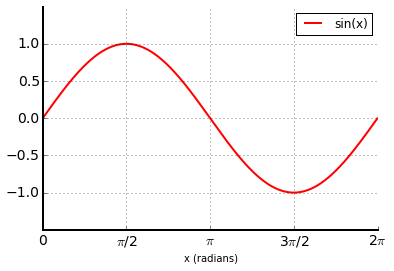First of all, we have modified the call to plt.plot() in order to decorate better the line. We have specified the line width and color according to the documentation.
Also, the axis should finish at 2*pi, since there is no graph after that. We can also increase the vertical range so that the maximum and minimum are not at the border of the graphic. We can do this by calling plt.axis(limits) in line 10. In line 11 we add a label for the x axis.
We then get a handle for the axes (line 13) to change the widths of the lower and left axes and hide the top and right ones (lines 15-18) by accessing each individual spine. We also modify each individual axis by placing the ticks (the little lines on the axes) on the visible axes (lines 20 and 21), setting the marks on significant values of the axes (22-24) and changing the font size (25). Note that whatever is written between \$ characters is interpreted as a Latex formula, which lets us include many math symbols. Finally, in order to make more easy the interpretation of the values, we add a grid where the major ticks are situated on each axis (27, 28). Hard and confusing; but this looks much better than the original:

In :
plt.figure()
plt.plot(x,y)
plt.legend(['sin(x)'])
plt.show()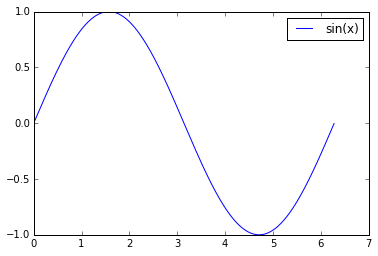Next, we will customize the barplot. Remember what it looked like:

In :
top_5 = ['USA','USSR','UK','France','China']
medals = [976, 395, 236, 202, 201]

plt.figure()
plt.bar(range(5),medals, align='center')
ax = plt.axes()
ax.set_xticks(range(5))
ax.set_xticklabels(top_5)
plt.show()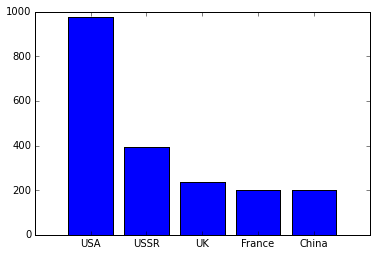Some customization has already been done, otherwise the default graphic would be completely useless. In this case, we may want to have each column painted in a different color, add some horizontal lines on the marks for the vertical axis, remove the ticks in the horizontal axis. Since the number of medals is not very readable by only observing the heights (look at France and China), we will add labels with the numbers on top of the bars. We will also remove the top, left and right axis lines.

In :
plt.figure()
plt.bar(range(5),medals, align='center', color=['blue','red','green','white','brown'], edgecolor='none')
ax = plt.axes()
ax.set_xticks(range(5))
ax.set_xticklabels(top_5)
ax.set_yticklabels([])

ax.set_axis_bgcolor('gold')

for i,n in enumerate(medals):
ax.text( i , n + 10, n, ha='center', va='bottom')

ax.spines['bottom'].set_linewidth(2)
ax.spines['left'].set_visible(False)
ax.spines['top'].set_visible(False)
ax.spines['right'].set_visible(False)

ax.xaxis.set_ticks_position('bottom')
ax.yaxis.set_ticks_position('none')

ax.yaxis.grid(True, which='major')
plt.axis([-1,5,0,1200])

plt.ylabel('Number of gold medals\nfor the top 5 countries')

plt.show()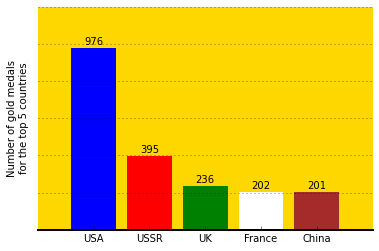Quick review: we modify line 2 in order to set a color for each column. If there are more columns than colors, the pattern will repeat again and again. In line 6 we remove all the y axis tick labels (the numbers). In line 8 we change the background color of the plot. In lines 10 and 11 we iterate over each value using enumerate() (to make the code clearer) and set a text label in position (i, n+10) where i is the index of the column (the x coordinate) and n is the number of medals (the y coordinate). The rest of the lines are similar to those used for the line plot used earlier.

Finally, we will modify the pie chart, that originally looks like this:

In :
browsers = ['Chrome', 'Firefox', 'IE', 'Safari', 'Opera']
users = [67.4, 19.2, 6.8, 3.9, 1.5]
browsers.append('Other')
users.append(round(100-sum(users),2)) # We use round to prevent a precission problem

plt.figure()
plt.pie(users,labels=browsers)
plt.show()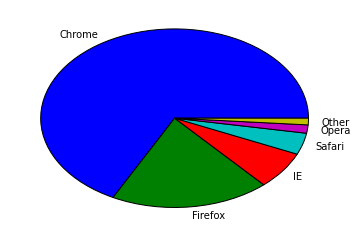Usually, the public will associate certain color with each browser. Using different colors will be confusing due to the Stroop Effect, that increases the brains reaction time when a color and the name associated to it (in this case, the name of the browser) do not match. Someone who saw the graphic above for half a second would think that it is greatly outdated since the most used browser seems to be IE (traditionally associated with blue). Only a closer look will reveal that it is actually Chrome.
So the first step would be to change the colors. Normally a pie chart is round; not the egg-looking eyesore represented above. We’ll fix that too. Finally, we’ll put all the labels in a single legend that also shows the percent of users of each browser.

In :
colors = ['yellow', 'orange', 'blue', 'cyan', 'red', 'gray']
legend = []
for browser, market in zip(browsers, users):
legend.append(browser + ' (' + str(market) + ' %)')

plt.figure()
plt.pie(users,colors=colors)
plt.axis('equal')
plt.title('Web browser market share in Nov. 2015', y=1.1, fontdict={'fontsize':20})
plt.legend(legend,bbox_to_anchor=(0, 1))
plt.show()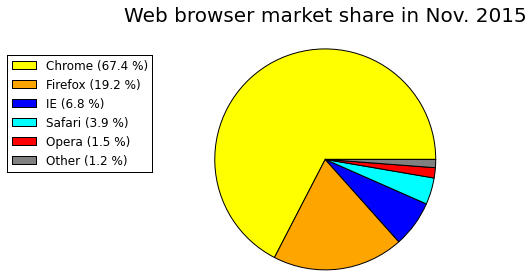In lines 3 and 4, we create each label of the legend by combining each entry of browsers and users by a for loop combined with a zip(). We removed the labels parameter from plt.pie() in line 7 so that the labels are not shown. In line 9 we add the title, specifying the font size and the height (so there is a margin between the title and the graphic). Finally, we paint the legend specifying its position as shown here.

Well, that’s all! Matplotlib has many graphic customization options. Here we have barely scratched the surface. But a very obvious fact is that, in order to obtain appealing graphics, we need to find a lot of obscure commands and settings. A whole book can be written on this subject. Anyway, the workflow with Matplotlib usually involves a lot of googling, and since it is a widely used library, the answer to almost any question can be found there.

This site uses Akismet to reduce spam. Learn how your comment data is processed.